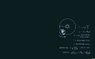# Calculating the moons mass

steven1495

## Homework Statement

A satellite is in circular orbit around the moon 250.0km above the moon's surface, and takes 2.000 hours and 14.00 minutes for one revolution of the moon. Calculate the moons mass.

The radius of the moon is 1737.4km, so if you add the extra 250.0km the satellite is above, the total radius is 1987.4km

Last edited:

SHISHKABOB
what sort of attempt have you made? You should have some formulas about centripetal force and the force due to gravity that you could use.

steven1495
Would you be able to use the equation in this picture? instead of solving for the time, which we could figure out easy enough, we solve for the mass, but instead of the sun, we change all the values to the ones for the moon, if you get my meaning

#### Attachments

•1305765361-earth-orbit-sun-365-physics-none-wallpaper.jpg
11.3 KB · Views: 399
SHISHKABOB
yes, that would work

steven1495
What does "μ" mean?

SHISHKABOB
it says there in the image

it's the gravitational constant G multiplied by the mass of what's being orbited. In the image, the sun is being orbited; in your problem, the moon is being orbited.

steven1495
Whats the gravitational constant?

SHISHKABOB
http://en.wikipedia.org/wiki/Gravitational_constant

do you recognize this equation:

$F = G\frac{Mm}{r^{2}}$ ?

I'm not sure why you'd be given a problem like this and not know that equation. The G in that equation is the gravitational constant.